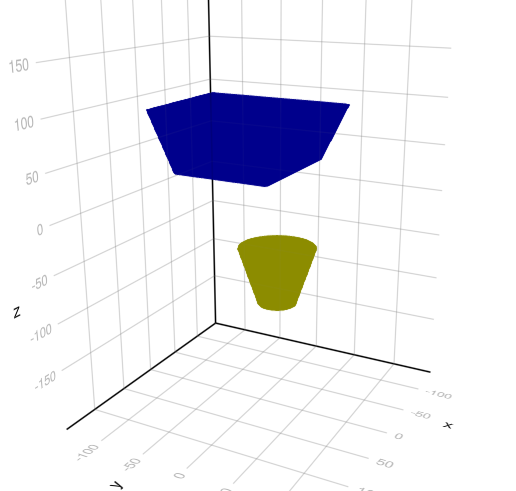# Getting 3D shapes without illumination

I am plotting with GLMakie my own 3D solids by defining `GeometryBasics.faces(...)` and `GeometryBasics.coordinates(...)` for each type. Then I plot the solids using a code like this:

``````   m = GeometryBasics.mesh(solid)
points = GeometryBasics.coordinates(m)
faces  = GeometryBasics.faces(m)
map!(c -> c * t, points, points)           # apply some transformation to the points
m = GeometryBasics.Mesh(points, faces)     # remake a mesh with the moved points
mesh!(s, m, color=color, transparency=true, visible=true)
``````

It works but I get the 3D shapes without illumination. Like this:Does anybody know what I should do to get a more realistic display of my solids?

This happens when you have missing or inverted normals. I always forget what the command is to get them but that might help you google itThis should work:

``````m = GeometryBasics.normal_mesh(solid)
points = GeometryBasics.coordinates(m)
faces = GeometryBasics.faces(m)
map!(c -> c * 0.1, points, points)           # apply some transformation to the points
m = GeometryBasics.Mesh(meta(points; normals=normals(m), faces))
``````

Thanks, but it does not work. Perhaps I am missing something else.
I am not able to construct a `normal_mesh` with my shapes, and therefore the `normals(m)` returns `nothing`.
I was able to construct a `mesh` because I had the following functions defined I guess:

``````    function GeometryBasics.Tesselation(s::G4VSolid, nvertices::NTuple{N,<:Integer}) where N
return Tesselation{3,Float64,typeof(s),N}(s, Int.(nvertices))
end
GeometryBasics.mesh(s::G4VSolid) = GeometryBasics.mesh(Tesselation(s, 64), facetype=typeof(first(GeometryBasics.faces(s))))
``````

How can I do the equivalent for `normal_mesh`? BTW, I do not find any documentation on `normal_mesh`.

I see…

That’s not really what you should overload - sorry, this is badly documented, and don’t have time right now to document itHave to look at e.g. Sphere to see what to overload.

Anyways, the easiest solution to get things working is to just do this:

``````m = GeometryBasics.Mesh(meta(points; normals=normals(points, faces), faces))
``````

Thanks very much. It works if I put the parenthesis in the right position

``````GeometryBasics.Mesh(meta(points; normals=normals(points, faces)), faces)
``````

I will have a look at the Sphere.